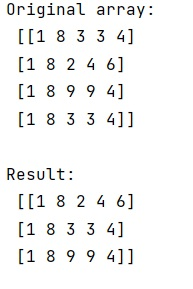# How to Remove Duplicate Elements from NumPy Array?

Learn, how to remove duplicate elements from NumPy array in Python?
Submitted by Pranit Sharma, on January 24, 2023

NumPy is an abbreviated form of Numerical Python. It is used for different types of scientific operations in python. Numpy is a vast library in python which is used for almost every kind of scientific or mathematical operation. It is itself an array which is a collection of various methods and functions for processing the arrays.

## Problem statement

Suppose we are given a 2-dimensional NumPy array that contains some repeated rows and we need to remove these repeated rows.

## Removing Duplicate Elements from NumPy Array

To remove a duplicate row, we will use numpy.unique() method. We will fetch each row in tuple form and pass it into this method as an argument. It will only return those rows which are not equal i.e., it will only return unique rows.

Let us understand with the help of an example,

## Python code to remove duplicate elements from NumPy array

```# Import numpy
import numpy as np

# Creating a numpy array
arr = np.array([
[1,8,3,3,4],
[1,8,2,4,6],
[1,8,9,9,4],
[1,8,3,3,4]])

# Display original array
print("Original array:\n",arr,"\n")

# Removing duplicate rows
new = [tuple(row) for row in arr]
res = np.unique(new,axis=0)

# Display result
print("Result:\n",res,"\n")
```

### Output

The output of the above program is: